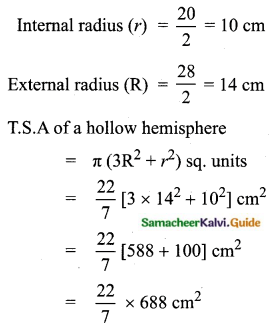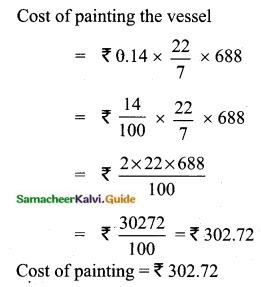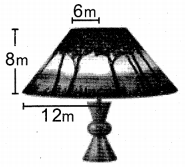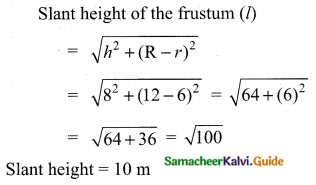Students can download Maths Chapter 7 Mensuration Ex 7.1 Questions and Answers, Notes, Samacheer Kalvi 10th Maths Guide Pdf helps you to revise the complete Tamilnadu State Board New Syllabus, helps students complete homework assignments and to score high marks in board exams.

## Tamilnadu Samacheer Kalvi 10th Maths Solutions Chapter 7 Mensuration Ex 7.1

Question 1.
The radius and height of a cylinder are in the ratio 5 : 7 and its curved surface area is 5500 sq.cm. Find its radius and height.
Let the radius be 5x and the height be 7x
C.S.A of a cylinder = 5500 sq.cm.
2πrh = 5500
2 × $$\frac{22}{7}$$ × 5x × 7x = 5500
2 × 22 × 5 × x2 = 5500
x2 = $$\frac{5500}{2 \times 22 \times 5}$$
x2 = 25 cm
x = 5 cm
Radius of the cylinder = 5 × 5 = 25 cm
Height of the cylinder = 7 × 5 = 35 cmQuestion 2.
A solid iron cylinder has total surface area of 1848 sq.m. Its curved surface area is five-sixth of its total surface area. Find the radius and height of the iron cylinder.
T.S.A of the cylinder =1848 sq.cm
2πr(h + r) = 1848 ……. (1)
Curved surface area = $$\frac{5}{6}$$ × 1848 sq.cm
2πrh = 5 × 308
2πrh = 1540 sq.m ……… (2)
Substitute the value of2πrh in (1)
2πr(h + r) = 1848
2πrh + 2πr2 = 1848
1540 + 2πr2 = 1848
2πr2 = 1848 – 1540
2 × $$\frac{22}{7}$$ × r2 = 308
r2 = $$\frac{308 \times 7}{2 \times 22}$$ = 49
r = 7
Radius of the cylinder = 7m
2πrh = 1540
2 × $$\frac{22}{7}$$ × 7 × h = 1540
h = $$\frac{1540}{2 \times 22}$$ = 35 m
Radius of the cylinder = 7 m
Height of the cylinder = 35 mQuestion 3.
The external radius and the length of a hollow wooden log are 16 cm and 13 cm respectively. If its thickness is 4 cm then find its T.S.A.
External radius of the wooden log (R) = 16 cm
Thickness = 4 cm
Internal radius (r) = 16 – 4 = 12 cm
Length of the wooden log (h) = 13 cm
T.S.A of the hollow cylinder = 2π (R + r) (R – r + h) sq.cm
= 2 × $$\frac{22}{7}$$ × (16 + 12) (16 – 12 + 13) sq.cm
= 2 × $$\frac{22}{7}$$ × 28 × 17 sq.cm
= 2 × 22 × 4 × 17 sq.cm.
= 2992 sq.cm.
T.S.A of the hollow wooden = 2992 sq.cm.Question 4.
A right angled triangle PQR where ∠Q = 90° is rotated about QR and PQ. If QR = 16 cm and PR = 20 cm, compare the curved surface areas of the right circular cones so formed by the triangle.In the Right Triangle
QP2 = PR2 – QR2= 202 – 162 = 400 – 256 = 144
QP = √144 = 12 cm
When PQ is rotated r = 12, l = 20
C.S.A of the cone = πrl sq. units = π × 12 × 20 cm2 = 240π cm2
When QR is rotated r = 16, l = 20
C.S.A of the cone = nrl sq. units = π × 16 × 20 = 320π cm2
C.S.A. of a cone when rotated about QR is larger.

Question 5.
4 persons live in a conical tent whose slant height is 19 cm. If each person requires 22 cm2 of the floor area, then find the height of the tent.
Slant height of a cone (r) = 19 cm
Floor area for 4 persons = 4 × 22 cm2
πr2 = 88 cm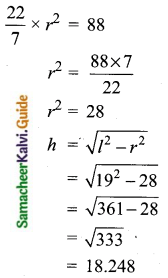Height of the tent = 18.25 cm

Question 6.
A girl wishes to prepare birthday caps in the form of right circular cones for her birthday party, using a sheet of paper whose area is 5720 cm2, how many caps can be made with radius 5 cm and height 12 cm.
Radius of a cap (r) = 5 cmQuestion 7.
The ratio of the radii of two right circular cones of the same height is 1 : 3. Find the ratio of their curved surface area when the height of each cone is 3 times the radius of the smaller cone.
Let the radius of the first cone be ‘x’ and the Height of the cone be 3xQuestion 8.
The radius of a sphere increases by 25%. Find the percentage increase in its surface area.
Let the radius of the be “r”
Surface area of the sphere = 4πr2 sq.units …….. (1)
If the radius is increased by 25%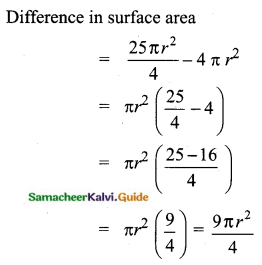Percentage of increase in surface area = 56.25 %

Question 9.
The internal and external diameters of a hollow hemispherical vessel are 20 cm and 28 cm respectively. Find the cost to paint the vessel all over at ₹ 0.14 per cm2.